# RD Sharma Solutions for Class 8 Maths Chapter - 7 Factorization Exercise 7.4

Students can download free pdf of RD Sharma Solutions for Class 8 Maths Exercise 7.4 Chapter 7 Factorization which is provided here. With utmost care, the solutions are designed by our expert faculty team at BYJU’S to help students understand the concepts clearly. Students are suggested to practice the solutions on a regular basis which helps in gaining knowledge and can attain good marks in their exams. Pdf’s of RD Sharma Solutions can be downloaded easily from the links given below.

This Exercise 7.4 of Chapter 7 Factorization is on based on the factorization by grouping the terms.

## Download the pdf of RD Sharma Solutions For Class 8 Maths Exercise 7.4 Chapter 7 Factorization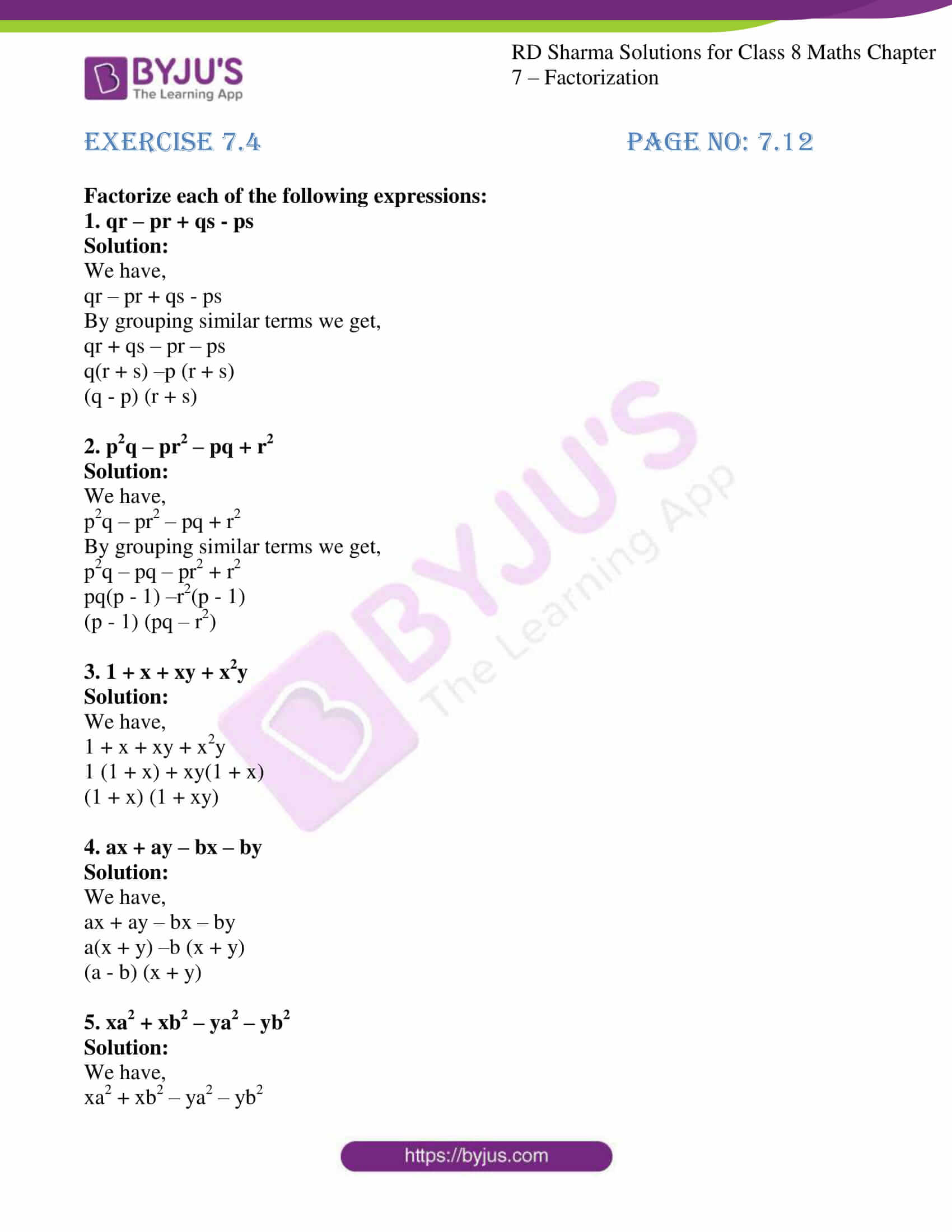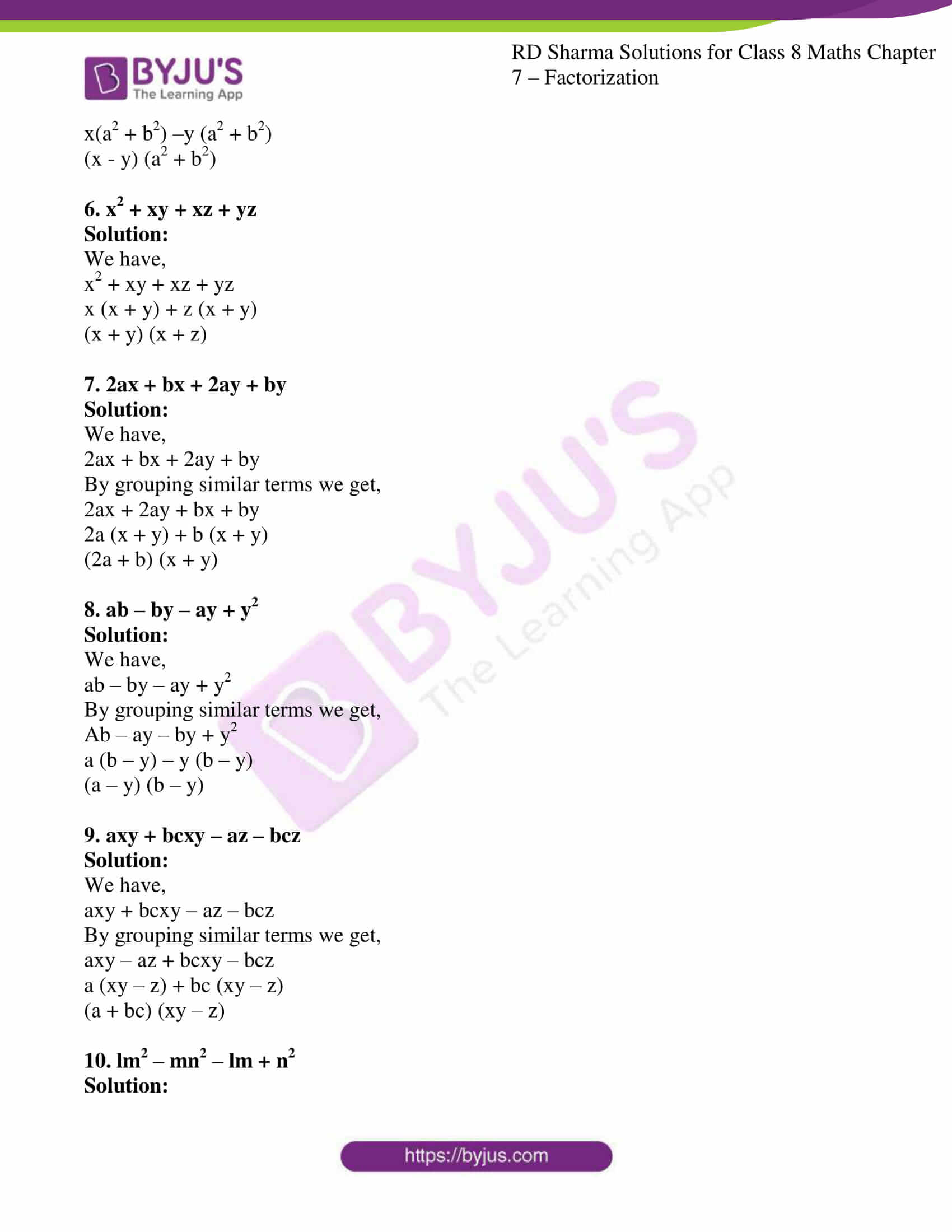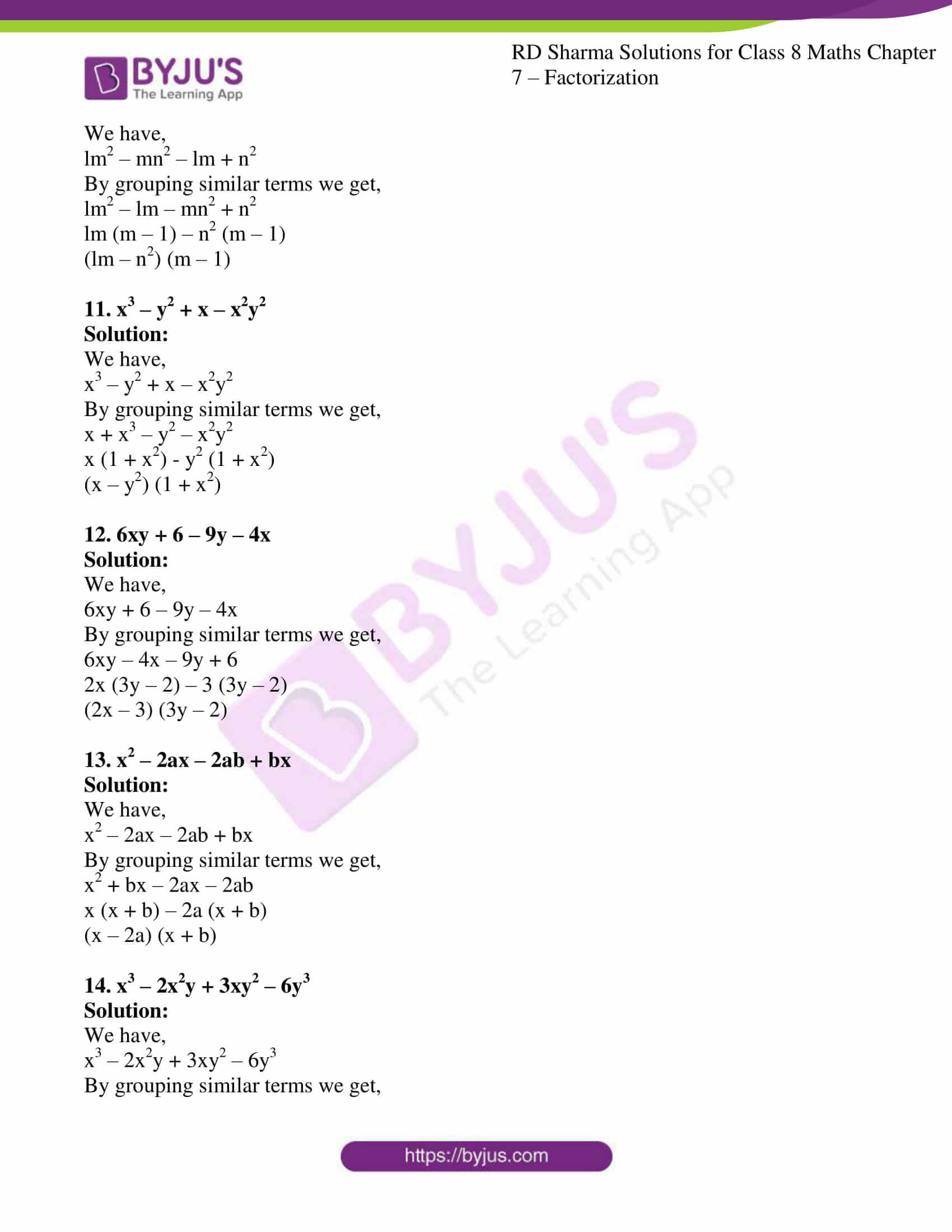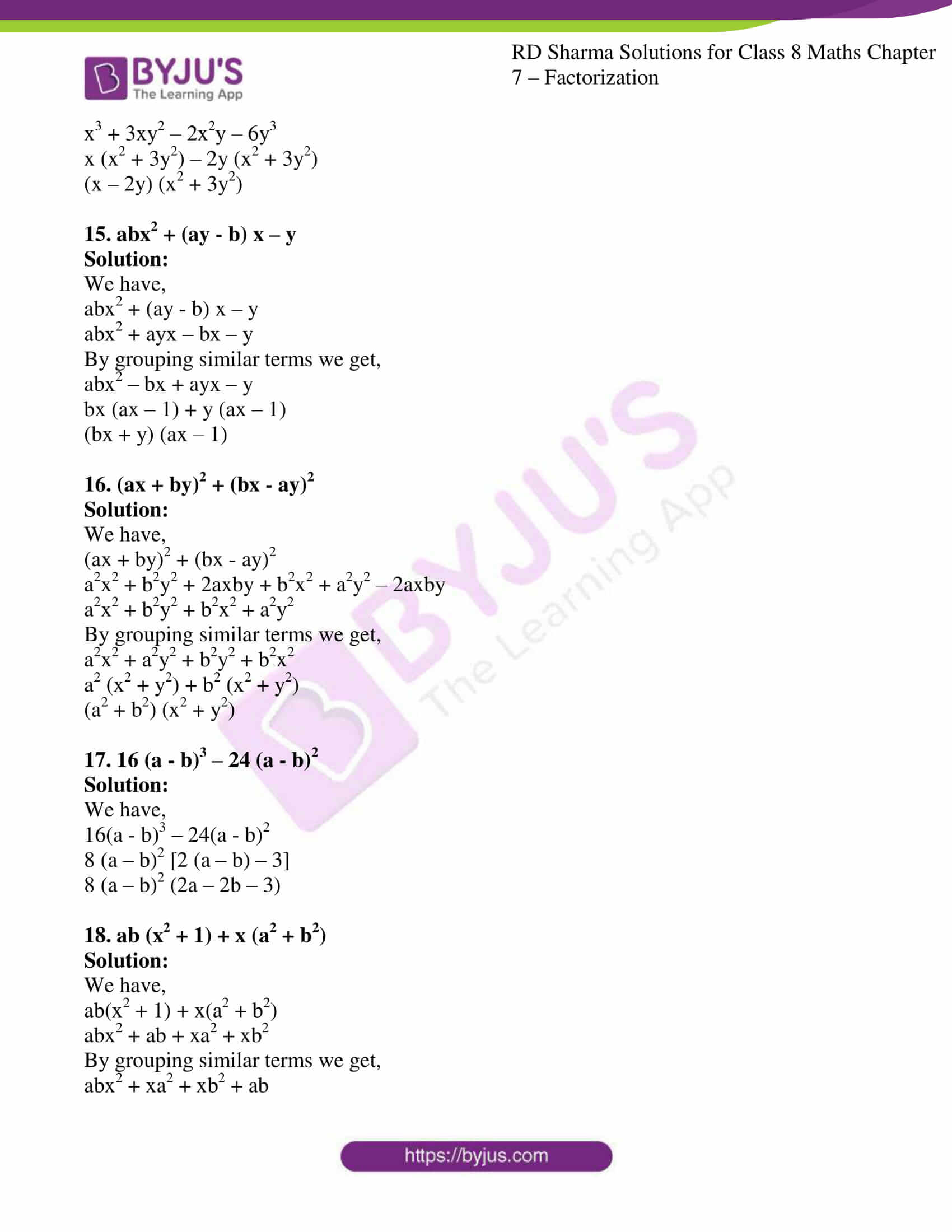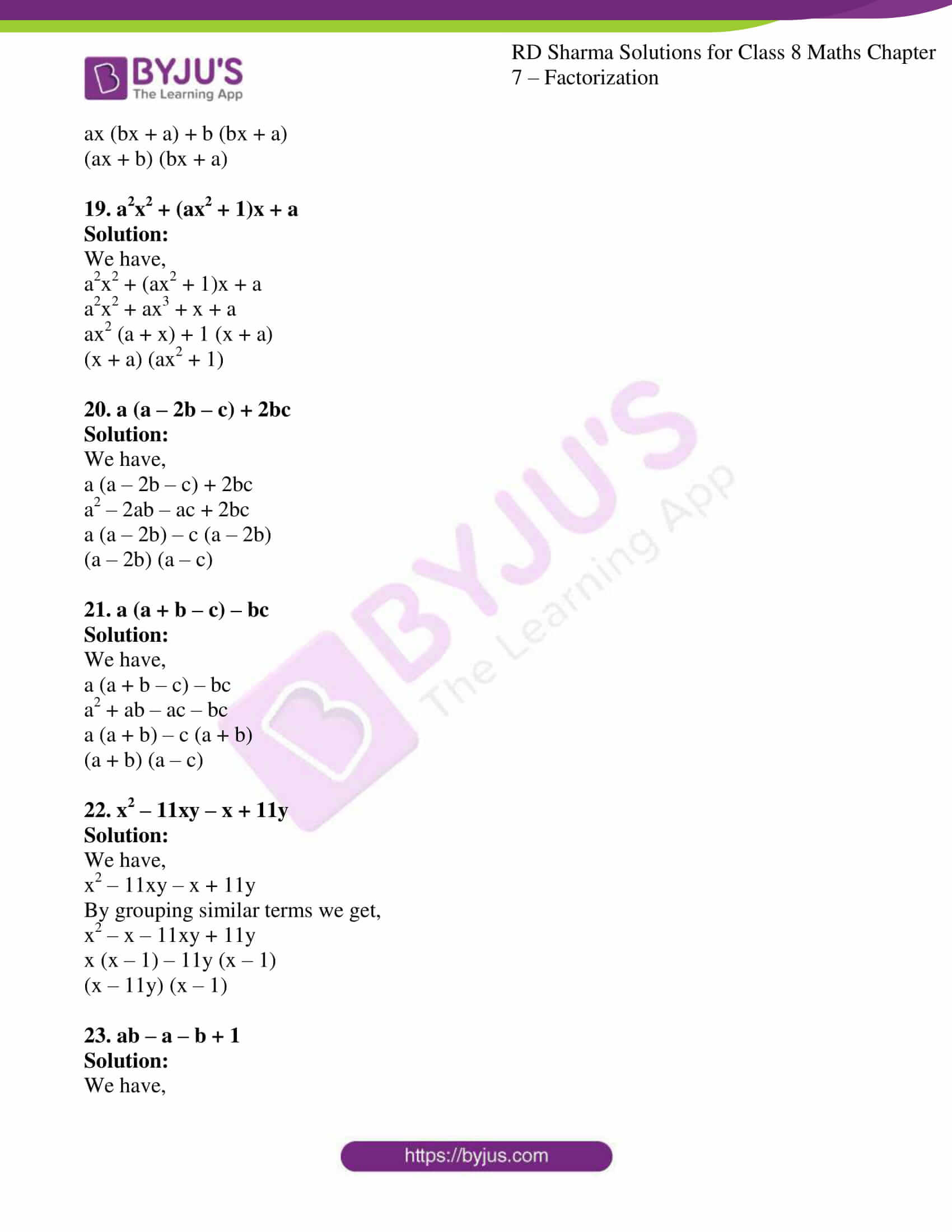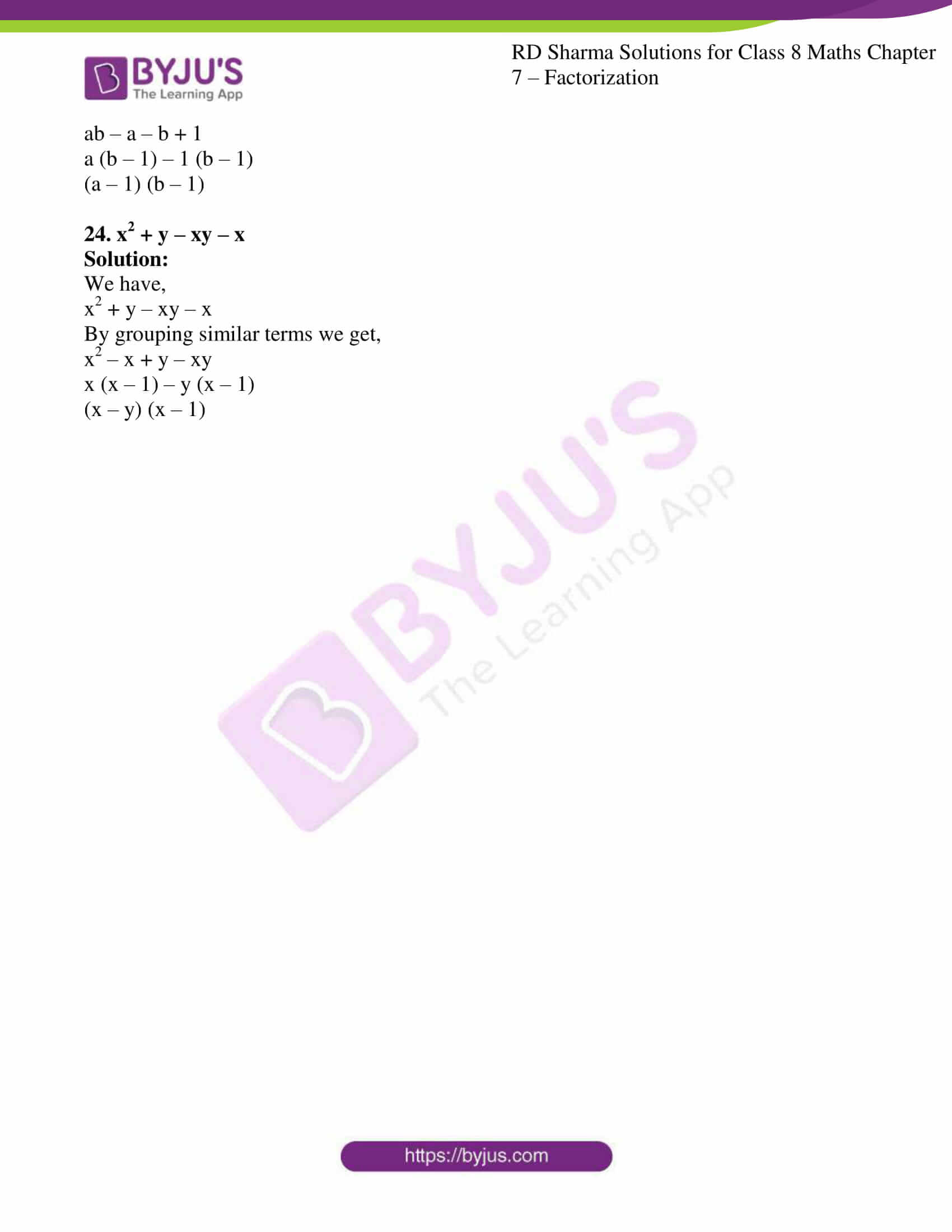### Access Answers to RD Sharma Solutions for Class 8 Maths Exercise 7.4 Chapter 7 Factorization

#### EXERCISE 7.4 PAGE NO: 7.12

Factorize each of the following expressions:

1. qr – pr + qs – ps

Solution:

We have,

qr – pr + qs – ps

By grouping similar terms we get,

qr + qs – pr – ps

q(r + s) –p (r + s)

(q – p) (r + s)

2. p2q – pr2 – pq + r2

Solution:

We have,

p2q – pr2 – pq + r2

By grouping similar terms we get,

p2q – pq – pr2 + r2

pq(p – 1) –r2(p – 1)

(p – 1) (pq – r2)

3. 1 + x + xy + x2y

Solution:

We have,

1 + x + xy + x2y

1 (1 + x) + xy(1 + x)

(1 + x) (1 + xy)

4. ax + ay – bx – by

Solution:

We have,

ax + ay – bx – by

a(x + y) –b (x + y)

(a – b) (x + y)

5. xa2 + xb2 – ya2 – yb2

Solution:

We have,

xa2 + xb2 – ya2 – yb2

x(a2 + b2) –y (a2 + b2)

(x – y) (a2 + b2)

6. x2 + xy + xz + yz

Solution:

We have,

x2 + xy + xz + yz

x (x + y) + z (x + y)

(x + y) (x + z)

7. 2ax + bx + 2ay + by

Solution:

We have,

2ax + bx + 2ay + by

By grouping similar terms we get,

2ax + 2ay + bx + by

2a (x + y) + b (x + y)

(2a + b) (x + y)

8. ab – by – ay + y2

Solution:

We have,

ab – by – ay + y2

By grouping similar terms we get,

Ab – ay – by + y2

a (b – y) – y (b – y)

(a – y) (b – y)

9. axy + bcxy – az – bcz

Solution:

We have,

axy + bcxy – az – bcz

By grouping similar terms we get,

axy – az + bcxy – bcz

a (xy – z) + bc (xy – z)

(a + bc) (xy – z)

10. lm2 – mn2 – lm + n2

Solution:

We have,

lm2 – mn2 – lm + n2

By grouping similar terms we get,

lm2 – lm – mn2 + n2

lm (m – 1) – n2 (m – 1)

(lm – n2) (m – 1)

11. x3 – y2 + x – x2y2

Solution:

We have,

x3 – y2 + x – x2y2

By grouping similar terms we get,

x + x3 – y2 – x2y2

x (1 + x2) – y2 (1 + x2)

(x – y2) (1 + x2)

12. 6xy + 6 – 9y – 4x

Solution:

We have,

6xy + 6 – 9y – 4x

By grouping similar terms we get,

6xy – 4x – 9y + 6

2x (3y – 2) – 3 (3y – 2)

(2x – 3) (3y – 2)

13. x2 – 2ax – 2ab + bx

Solution:

We have,

x2 – 2ax – 2ab + bx

By grouping similar terms we get,

x2 + bx – 2ax – 2ab

x (x + b) – 2a (x + b)

(x – 2a) (x + b)

14. x3 – 2x2y + 3xy2 – 6y3

Solution:

We have,

x3 – 2x2y + 3xy2 – 6y3

By grouping similar terms we get,

x3 + 3xy2 – 2x2y – 6y3

x (x2 + 3y2) – 2y (x2 + 3y2)

(x – 2y) (x2 + 3y2)

15. abx2 + (ay – b) x – y

Solution:

We have,

abx2 + (ay – b) x – y

abx2 + ayx – bx – y

By grouping similar terms we get,

abx2 – bx + ayx – y

bx (ax – 1) + y (ax – 1)

(bx + y) (ax – 1)

16. (ax + by)2 + (bx – ay)2

Solution:

We have,

(ax + by)2 + (bx – ay)2

a2x2 + b2y2 + 2axby + b2x2 + a2y2 – 2axby

a2x2 + b2y2 + b2x2 + a2y2

By grouping similar terms we get,

a2x2 + a2y2 + b2y2 + b2x2

a2 (x2 + y2) + b2 (x2 + y2)

(a2 + b2) (x2 + y2)

17. 16 (a – b)3 – 24 (a – b)2

Solution:

We have,

16(a – b)3 – 24(a – b)2

8 (a – b)2 [2 (a – b) – 3]

8 (a – b)2 (2a – 2b – 3)

18. ab (x2 + 1) + x (a2 + b2)

Solution:

We have,

ab(x2 + 1) + x(a2 + b2)

abx2 + ab + xa2 + xb2

By grouping similar terms we get,

abx2 + xa2 + xb2 + ab

ax (bx + a) + b (bx + a)

(ax + b) (bx + a)

19. a2x2 + (ax2 + 1)x + a

Solution:

We have,

a2x2 + (ax2 + 1)x + a

a2x2 + ax3 + x + a

ax2 (a + x) + 1 (x + a)

(x + a) (ax2 + 1)

20. a (a – 2b – c) + 2bc

Solution:

We have,

a (a – 2b – c) + 2bc

a2 – 2ab – ac + 2bc

a (a – 2b) – c (a – 2b)

(a – 2b) (a – c)

21. a (a + b – c) – bc

Solution:

We have,

a (a + b – c) – bc

a2 + ab – ac – bc

a (a + b) – c (a + b)

(a + b) (a – c)

22. x2 – 11xy – x + 11y

Solution:

We have,

x2 – 11xy – x + 11y

By grouping similar terms we get,

x2 – x – 11xy + 11y

x (x – 1) – 11y (x – 1)

(x – 11y) (x – 1)

23. ab – a – b + 1

Solution:

We have,

ab – a – b + 1

a (b – 1) – 1 (b – 1)

(a – 1) (b – 1)

24. x2 + y – xy – x

Solution:

We have,

x2 + y – xy – x

By grouping similar terms we get,

x2 – x + y – xy

x (x – 1) – y (x – 1)

(x – y) (x – 1)